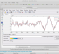## Kalman Filter for Speech DenoisingStarted by 3 years ago2 replieslatest reply 3 years ago194 views

Hi, I have been trying to denoise a speech signal using Kalman Filter. I have use the following code for my calculation. May I know if I am in the right direction? Many literature that I have read uses a state space model for the Kalman Filter? How can I do that for a corrupted speech signal? System Identification?

Thanks.

def my_calculation(y_corrupt):

sum1 = 0

square1 = 0

mean1 = 0

E_estimated = 0.00001

E_measured = 0.00001

noise_model= np.zeros((len(y_corrupt)))

y_filtered = np.zeros((len(y_corrupt)))

N = len(y_corrupt)

for i in range (2,N):

KG = E_estimated/(E_estimated+E_measured)

#KG = KG**2

noise_model[i] = noise_model[i-1] + KG*(y_corrupt[i]-noise_model[i-1])

E_estimated= (1-KG)*E_measured;

#E_estimated= (1-KG)*E_estimated;

square1 = square1 + noise_model[i]**2

sum1 = sum1 + noise_model[i]

mean1 = (mean1*(i-1)+noise_model[i])/i

E_measured = square1/i - mean1**2

y_filtered[i] = y_corrupt[i] - noise_model[i]

return y_filtered

[ - ]